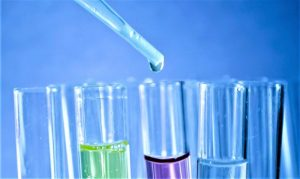Themes

# What is the limiting reagent?

## Limiting reagent

In the field of chemistry we know by the name of reagent the substance that is responsible for generating a chemical reaction . It is a substance that allows to show the presence of a different substance which, through an interaction , can generate a new product . Reagents are then chemical elements that are responsible for establishing an interaction with other substances in a chemical reaction, resulting in a substance with different properties that is called a product . There are several types of reagents, in this case, we will explain the limiting reagent .## What is the limiting reagent?

In a chemical reaction the limit reagent is the substance which consumes so complete when given a particular chemical reaction , and that limits the amount of product formed.

• Definition
• How to calculate the limiting reactant
• What is the limiting reagent for?
• Example

## Definition

Many times, when a chemical reaction is generated in the laboratory, one of the reactants that reacts during the process will always react completely so that the chemical equation can be balanced with its corresponding stoichiometric coefficients , in this case we refer to the limiting reagent. The limiting reagent is then the substance that is consumed first and which limits the formation of other products.

The limiting reagent is the reagent that is completely consumed when a reaction occurs and, therefore, is the one in charge of determining when the reaction stops . From the stoichiometry of the reaction, the exact amount of reactant that is needed to react with another element can be calculated . If the reactants are not mixed in the correct stoichiometric ratios, in other words, in the way as indicated by the chemical equationbalanced, then one of the reagents will be completely consumed, while another will not be able to. The limiting reagent is the one that is totally consumed; prevents the reaction from continuing because there is nothing left to react with the excess reagent .

In order to identify which is the limiting reagent within a chemical equation, the following two methods can be used :

• Probing : through this process, one of the reactants is chosen as the limiting assumption and this will then be the one that reacts absolutely or completely . Then the necessary amount of the other reagent must be calculated and we check that it is less than the mass that we have. In case of not having enough, since we cannot spend more than we have, the limiting reagent will be the other.
• Proportion : the proportion in grams or in moles in which the reactants react must be calculated and compared with the data. We study which one we should have more of so that the stoichiometric relationship is fulfilled and this is the limiting factor.

## How to calculate the limiting reactant

The way to calculate the limiting reactant is using the following equation:

aX + bY → cZ

equation in which:

• X and Y are the reactants
• Z are the products
• a, b and c are the respective stoichiometric coefficients

Yes:

mol X available / mol Y available <a / b

So X will be the limiting reagent.

Another way to find the limiting reactant would be by applying the following steps:

• Determine the balanced chemical equation for the chemical reaction under study.
• All given information must be converted into moles (most likely, through the use of molar mass as a conversion factor).
• Calculate the molar ratio from the given information. The calculated ratio must be compared to the actual ratio.
• Use the amount of limiting reagent to calculate the amount of product produced .
• If necessary, calculate how much excess non-limiting reagent is left.

The third way to find the limiting reactant:

• Balance the chemical equation for the chemical reaction .
• Convert the given information into moles .
• Use stoichiometry for each individual reagent to find the mass of the product produced.
• The reagent that produces the least amount of product is the limiting reagent .
• The reagent that produces the most product is the excess reagent .
• To find the amount of excess of remaining reagent , subtract the mass of excess reagent consumed of the total mass of the reagent in excess given .

## What is the limiting reagent for?

The limiting reagent is the one in charge of delimiting the amount of product that can be formed in a chemical reaction . It is the one that is in charge of limiting the possibility that a reaction has of being able to continue or follow its course, so at the same time, it is in charge of limiting the amount of the product that is produced by the reaction . So, we can say that the limiting reactant stops the reaction.

## Example

Let the synthesis reaction of methanol be:  CO  + 2 2 → C 3 OH .

If you have 3 moles of CO and 5 moles of, calculate the limiting reagent and the amount of methanol produced.

We calculate which is the limiting reactant:

• b moles of   = 2 3  =  6
•  moles of   = 1 · 5  =  5

Since b moles X> a moles Y  →  Y  is the  limiting reactant ,  that is,  2

The amount of methanol that is formed will therefore be:

• moles of Z = moles of Y · (c / b) = 5 · (1/2) =  2.5 moles of methanol